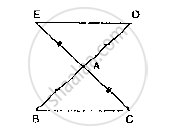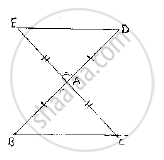Share
Notifications

View all notifications
Books Shortlist
Your shortlist is empty

# In Fig. 10.22, the Sides Ba and Ca Have Been Produced Such That: Ba = Ad and Ca = Ae. Prove that Segment De || Bc. - CBSE Class 9 - Mathematics

Login
Create free account

Forgot password?
ConceptCongruence of Triangles

#### Question

In Fig. 10.22, the sides BA and CA have been produced such that: BA = AD and CA = AE.
Prove that segment DE || BC.#### Solution

Given that, the sides BA and CA have been produced such that BA =AD  and CA= AE  and
given to prove  DE || BC
Consider triangle BAC and , DAE
We have
BA = ADand CA = AE [∵ given in the data]
And also ∠BAC=∠DAE [ ∵vertically opposite
angles]
So, by SAS congruence criterion, we have ΔBAC ≅ ΔDAE
⇒ BC = DE and ∠DEA=∠BCA, ∠EDA ∠CBA
[Corresponding parts of congruent triangles are equal]
Now, DE and BC are two lines intersected by a transversal DB such that ∠DEA =∠BCA,
i.e., alternate angles are equal
Therefore, DE || BCIs there an error in this question or solution?

#### APPEARS IN

Solution In Fig. 10.22, the Sides Ba and Ca Have Been Produced Such That: Ba = Ad and Ca = Ae. Prove that Segment De || Bc. Concept: Congruence of Triangles.
S